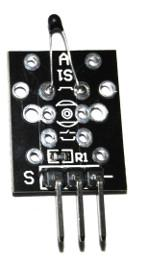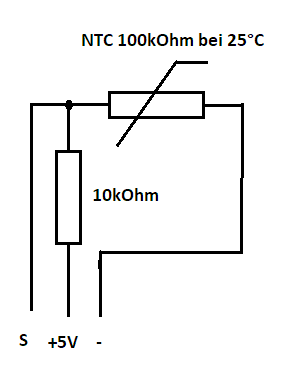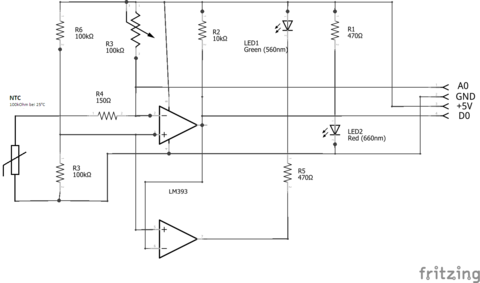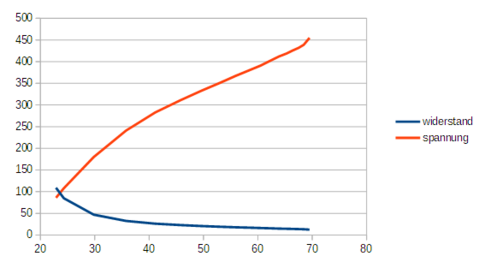The set contains two such modules. Both modules use the same hot conductor. One of these, however, also has a compensator that sets the digital output to zero depending on the potentiometer setting at a certain temperature. Amm Analog output is practically the same signal as the simple sensor.

Simple sensorSensor with CompactorCharacteristics

The NTC is a resistance that lowers the resistance value when heated. To measure temperatures with the NTC and not only to switch at a certain temperature, you need the characteristic. The diagram shows the resistance value in kOhm (blue) and the (1024) starting value read with analogRead (red);You can see through the used voltage divider the output voltage is partially linear.

Determination of a correction curve:

With a simple circuit we can try to determine a correction curve. For this we use the simple hot conductor sensor and the DS18B20 as a reference. We connect the middle pins of both modules with +5V at Arduino and the pins of both modules with GND. We connect the S-pin of the NTC module with A0 and the S-pin of the DS18B20 module with D2. To get the same temperature for both sensors we connect them as shown in the following picture.Now we download the following program to the Arduino. And connect the pin 8 at Arduino to Ground to stop the output.

/* This program determines the correction curve for an NTC
*Heat conductor and then tests it
*A DS18B20 sensor is used as a reference
*/

'35; include <OneWire.h>
'35; include <DallasTemperature.h>

//Connections
const byte ds18b20 = 2; //sensor reference
const byte sensor = 0; //to Eating Sensor
const byte output = 8; // Input to suppress output.
const byte point = 13; //measured

//instances for reference sensor DS18B20
OneWire oneWire(ds18b20);
DallasTemperature sensors(&oneWire);

//for storing correction data we create our own class
class Korr
public:
int minval; //Initial validity value
int maximum choice; //Final value of validity
float factor; //Correction
float delta; //relative offset

floating temperature(int choice)
float temp = float(val) * factor + delta;
return temp
}
};

//global variables
Korr curve ; //Array with correction values
byte number = 0; //Number of existing correction values

byte measurement = 1;
float should = 0; //current target value
int meaning = 0; //current sensor value

float should be old = 0; //last set value
float senseu alt = 0; //last sensor value

void setup()
//start rope interface
Serial.start(115200);
//Prepare reference sensor
sensors.start();
// Solution to 10 bit;
//output for led
pinMode(led, OUTPUT);
pinMode(output, INPUT)
measurement = 1; //we are in measuring mode
//once measurement for start values
Serial.println("Start Analysis");
measured();
should be used;
meaning of other meaning;
}

//function for reading the measured values
void measurements(){
//Request reference value
sensors.requestTemperatures();
//as the voltage decreases with increasing temperature
//we reverse the value by deducting it from the maximum value
}

void loop()
//Show state
digitalWrite(led,measurement);
//determine values
measured();
if (measurement) {
//we check whether we have reached a certain difference
//Subsection all 5oC
if ((should-be old) >= 5)
Serial.print("Calculation for");
Serial.print(shall);
Serial.println("C");
curve[number].minval = senseu alt;
curve[number].maxval = senses;
float factor =(should-u-old)/float(senses-u-old);
curve[number].factor = factor;
curve[number].delta = shall -(meaning * factor);
should be used;
meaning of other meaning;
number+;
}
if (should > 50)
measurement = 0;
Serial.println("analysis completed");
for (byte i = 0; i<number; i+) {
Serial.print(curve[i].mineval);
Serial.print("-)
Serial.print(curve[i].maxval);
Serial.print("-)
Serial.print(curve[i].factor);
Serial.print("-)
Serial.println(curves[i].delta);
}
delay(5000);
}
} else {
bytes in = 0;
if (sense > curve.minval) {
while ((senses > curve[i].maxval) && (i<number)) i++;
}
{
/* Helpful for checking the function
Serial.print("Found: Min =");
Serial.print(curve[i].mineval);
Serial.print("Max=");
Serial.print(curve[i].maxval);
Serial.print("Fact=");
Serial.print(curve[i].factor);
Serial.print("Delta=");
Serial.print(curves[i].delta);
Serial.print("Feeder=");
Serial.println(senses);
*/

float is = curve[i].temperature(senses);
Serial.print("Measurement values: Soll =");
Serial.print(shall);
Serial.print("Is=");
Serial.print(ist);
Serial.print("Deviation=");
Serial.println(soll-ist);
}
delay(1000);
}
}

After startup, the program is in analytical mode, i.e. whenever the temperature has risen by five degrees, the rise and the offset are calculated and stored in a table. This occurs until the temperature exceeds 50oC.

The easiest way to achieve temperature change is with a hairdryer. However, care must be taken to ensure that heating does not occur too quickly, as the two sensors have different inertia.

When a temperature of 50oC is reached, the content of the correction curve is displayed on the serial monitor. From now on, the target value, the actual value and the deviation can be displayed. Since the display has been suppressed (pin 8) to view the results of the analysis, it is necessary to separate the pin 8 from GND.

In my experiments I was not satisfied with the result. The temperature change was perhaps too rapid during the analysis.

Have fun experimenting.